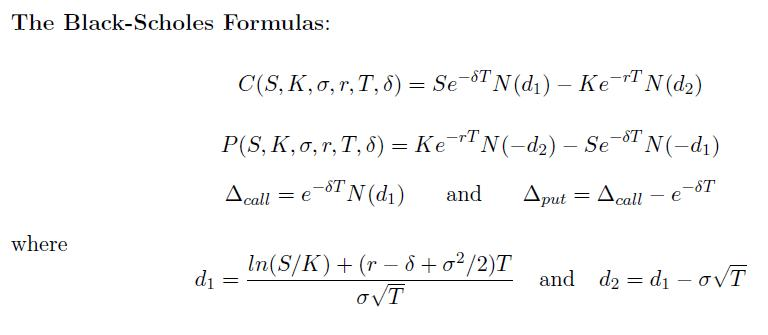FreqOn: ?→A: ?→B: ?→C: ?→D:  C ÷ E 2→C: D ÷ E 2→D: ?→X: ?→Y: X , Y ÷ E 2 ; A DT:

( ln( n÷B ) + ( D - maxY + C2 ÷ 2) maxX ) ÷ ( C√( maxX→A:

Ans - C√( maxX→M: 0→X: Lbl 2: C→Y: 1 ÷ (1 + . 231642Abs( A:

E - 7 √( e^( - A2 ) )( 1274148Ans - 1422484Ans2 + 7107069Ans3 -

7265760Ans2 2 + 5307027Ans^( 5→C: A>0 => 1 - C→C: M→A:

X=0→X: Ans => Goto 2: Ye^( - maxY maxX→A◢ Ans - e^( - maxY maxX

nA - BCe^( - DmaxX◢ Ans - ne^( - maxY maxX ) + B ÷ e^( maxX D

EXE (顯示Δput為 - 0.18465677) EXE (顯示C為10.1265606) EXE (顯示P為1.809343554)